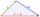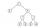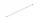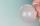# Triangle ABC

In a triangle ABC side b measure 10 cm less than the side a and side b is half of the side c. Calculate the length of sides if the circumference of the triangle is 42 cm.

Result

a =  18
b =  8
c =  16

#### Solution:

a+b+c=42
b = a-10
b = c/2

a+b+c = 42
a-b = 10
2b-c = 0

a = 18
b = 8
c = 16

Calculated by our linear equations calculator.

Leave us a comment of example and its solution (i.e. if it is still somewhat unclear...):Be the first to comment!#### To solve this example are needed these knowledge from mathematics:

Do you have a system of equations and looking for calculator system of linear equations? See also our trigonometric triangle calculator.

## Next similar examples:

1. TriangleDetermine if it is possible to construct a triangle with sides 28 31 34 by calculation.
2. TriangleProve whether you can construct a triangle ABC, if a=9 cm, b=10 cm, c=4 cm.
3. RectangleThe length of one side of the rectangle is three times the length of the second side. What are the dimensions of the rectangle if its circumference 96 cm?
4. RectangleCalculate perimeter of the rectangle with sides a=2.4 m and b=1.9 m.
5. BenchesThe park has 64 benches. Occupied are by 18 more than empty. How many benches are occupied and empty ?
6. One frame5 picture frames cost € 12 more than three frames. How much cost one frame?
7. Garden plotCalculate how many meters of fence need to fence the square garden with length and width of 22 meters.Added together and write as decimal number: LXVII + MLXIV
9. Eq1Solve equation: 4(a-3)=3(2a-5)
10. Math classificationIn 3A class are 27 students. One-third got a B in math and the rest got A. How many students received a B in math?
11. ExpressionsFind out value of expressions if a = -1, b =2: x=b - 2a - ab y=a3 - b2 - 2ab z=a2 b3 - a3 b2 w=a + b + a3 - b2
12. Valid numberRound the 453874528 on 2 significant numbers.
13. The resultHow many times I decrease the number 1632 to get the result 24?
14. LineHow many parts of line divide 5 (different) points that lie on it?
15. If-then equationIf 5x - 17 = -x + 7, then x =
16. Ten pupils10 pupils went to the store. 6 pupils bought lollipops and 9 pupils bought chewing gum. How many pupils have bought both lollipops and chewing gums (if everyone bought something)?
17. Six te 2If 3t-7=5t, then 6t=